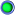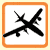Thread: Improved Search Function View Single Post
#200
 nfc5382X-Adept Join Date: Nov 2002 Posts: 475Quote:
Originally Posted by Grant
Quote:
 Originally Posted by taltos1 Excellent post folks, but any idea on 4.0.18, I saw that 4.0.16 seems to be working (with a post from page 12 or 12) but does anyone have this up and running 4.0.18? Thanks a lot

I'd like to know this too before trying to install this mod.

This is for 4.0.18. It also searches the productid by default. Both admin and customers constantly search by the product id!

find:
Code:
`# Search for substring in some fields...`

replace with:
Code:
```    # Search for substring in some fields...

\$ss = split(" ",\$data["substring"]);
\$ss_condition = "";
foreach(\$ss as \$s)
{
\$condition = array();

if (!empty(\$data["by_title"])) {
if(\$current_area == 'C' || \$current_area == 'P') {
\$condition[] = "IF(\$sql_tbl[products_lng].product IS NOT NULL AND \$sql_tbl[products_lng].product != '', \$sql_tbl[products_lng].product, \$sql_tbl[products].product) LIKE '%".\$s."%'";
} else {
\$condition[] = "\$sql_tbl[products].product LIKE '%".\$s."%'";
}
}

if (!empty(\$data["by_shortdescr"])) {
if(\$current_area == 'C' || \$current_area == 'P') {
\$condition[] = "IF(\$sql_tbl[products_lng].descr IS NOT NULL AND \$sql_tbl[products_lng].descr != '', \$sql_tbl[products_lng].descr,  \$sql_tbl[products].descr) LIKE '%".\$s."%'";
} else {
\$condition[] = "\$sql_tbl[products].descr LIKE '%".\$s."%'";
}
}

if (!empty(\$data["by_fulldescr"])) {
if(\$current_area == 'C' || \$current_area == 'P') {
\$condition[] = "IF(\$sql_tbl[products_lng].full_descr IS NOT NULL AND \$sql_tbl[products_lng].full_descr != '', \$sql_tbl[products_lng].full_descr, \$sql_tbl[products].fulldescr) LIKE '%".\$s."%'";
} else {
\$condition[] = "\$sql_tbl[products].fulldescr LIKE '%".\$s."%'";
}
}

## always search productid
if(\$current_area == 'C' || \$current_area == 'P') {
\$condition[] = "IF(\$sql_tbl[products_lng].productid IS NOT NULL AND \$sql_tbl[products_lng].productid != '', \$sql_tbl[products_lng].productid, \$sql_tbl[products].productid) LIKE '%".\$s."%'";
} else {
\$condition[] = "\$sql_tbl[products].productid LIKE '%".\$s."%'";
}
## //always search productid

if (!empty(\$data["extra_fields"]) && \$active_modules['Extra_Fields']) {
foreach(\$data["extra_fields"] as \$k => \$v) {
\$condition[] = "(\$sql_tbl[extra_field_values].value  LIKE '%".\$s."%' AND \$sql_tbl[extra_fields].fieldid = '\$k')";
}
\$join['extra_field_values'] = "\$sql_tbl[products].productid = \$sql_tbl[extra_field_values].productid";
\$join['extra_fields'] = "\$sql_tbl[extra_field_values].fieldid = \$sql_tbl[extra_fields].fieldid AND \$sql_tbl[extra_fields].active = 'Y'";
}

if (!empty(\$condition)) {
\$ss_condition .= " AND (".implode(" OR ", \$condition).")";
}
}

\$search_condition .= \$ss_condition;

# Search statistics
if (\$page == '0') {
db_query("INSERT INTO \$sql_tbl[stats_search] (search, date) VALUES ('\$data[substring]', '".time()."')");
}
} # /if (!empty(\$data["substring"]))

#
# Search by product features
#```

until:
Code:
```#
# Search by product features
#```
__________________
-----------------------
x-cart v4.7.6 [LIVE]
x-cart v4.0.18 [retired 2004-2016]
x-cart v3.5.13 [retired]
x-cart v3.4.14 [retired]